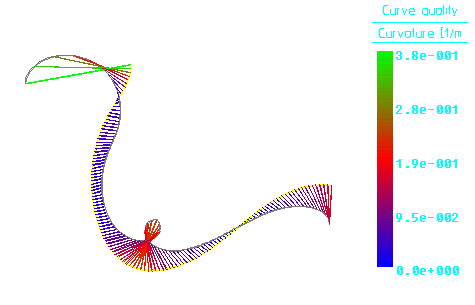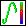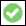> > Analyze a curve

Analyze a curve
In Creo Elements/Direct Modeling, surfaces are constructed from curves. It is, therefore, important to avoid deficiencies in curves to ensure a better quality of surface. To this end, Creo Elements/Direct Modeling provides a CurvatureAnalysis utility to help you during the early design stage of complex surfaces.
The CurvatureAnalysis utility uses vectors to represent the curvature at points on a curve. The vector length corresponds to the curvature value of the curve at that point, and its direction corresponds to the normal direction of the curve at that point. Since the curvature value is the reciprocal of the curvature-circle radius, the unit used in the analysis is 1/length unit.
Color-coding, which you can adjust, is used to aid the visual interpretation of curvature values.
An analysis can illustrate the following information:
High curvature values correspond to small radii in the curve, which are displayed by relatively long vectors in an analysis.
Wiggling curves (those with curvature changing from convexity to concavity) are recognized by the outline of the curvature field often crossing the curve itself.
The torsion of a curve is evaluated by noting how fast the curvature field rotates around the curve.
The quality of the parametrization can also be evaluated. In well-parametrized curves, the distance between two vectors is evenly spaced; ill-parametrized curves display an uneven distribution of vectors.
You can control two parameters used to analyze the curvature. The vectors can be scaled; for example, to amplify small effects on a curve. You can also halve or double the number of points on the curve at which its curvature is evaluated. These adjustments are made dynamically as you view an analysis.Creo Elements/Direct Modeling uses a color distribution (for example, Green-Red-Blue) to display the analysis result. The largest curvature values are displayed in the first color (Green), the middle color (Red) represents medium curvature, and the last color (Blue) represents low curvature. All values smaller than the negative limit or greater than the positive limit are displayed with the most intense color values. Between the limits the color values are interpolated with respect to the current curvature value.
To analyze curvature,
1. Click Analysis and then, in the Analyze group, clickCurve. The Crv Analysis dialog box opens.
2. Click on the edges to analyze.
3. Adjust the appearance of the analysis as required, using the Scaling, Density, and Colors options.
4. Continue to adjust analysis parameters, or clickto end the curvature analysis.